### MATH 1280 Introduction to Statistics – Assignment Unit 4 – Part 2

Abstract, painted by Jackson Pollock

A Random Variable:
9. The missing probability value (under the number 4) in the random variable table above is: 0.15
1 - (0.10+0.15+0.25+0.10+0.10+0.15) = 0.15

10. The sum of the probabilites in the second row of any random variable table like the one above should equal (round to 3 decimal places): 1
Based on theory that for any Random Variables, sum of probabilities should be 1 (one)

11. Read section 4.4.1 in the book (Yakir, 2011).  Do the numbers in the table above (for the random variable) represent a data sample (Yes/No)? No
It is because the Table above represents the sample space.

Painted by Jackson Pollock

12. In the random variable table shown above, the value in the second row represents the cumulative probability of the corresponding values in the first row (True/False) : FALSE
It is because the row represents the individual probability for each of the sample space. the probability value for the last entry is 1.

13. The probability that a randomly selected value from this random value will be less than or equal to 3 is : 0.6
Steps to find answer: P(X<=3) = 0.10+0.15+0.25+0.10 = 0.60

14. What is the probability that a randomly selected value from the random variable would be exactly 1.5?

15. Review section 4.4 in the book (Yakir, 2011), especially pages 57—58.  The expectation of the random variable is: 2.95
E(X) = 0*0.10 + 1*0.15 + 2*0.25 + 3*0.10 + 4*0.15 + 5*0.10 + 6*0.15 = 2.95

Painted by Jackson Pollock

16. To find the expectation of a random variable by using a relative frequency table, you can add the values in the first row of the table and divide by the number of columns in the table (True/False): FALSE
Steps to find answer: We use formula to find the expectation of a random variable as of to answer question 15

17. Study Yakir (2011) pp. 57-59 and solved problems 4.1.6-4.1.8.  The (population) standard deviation of the random variable above is (round to 3 decimal places): 1.909
(hint, you can not put values from the table into the sd() function because the sd() function does not adjust for the probabilities).
E(X^2) = 0*0.10 + 1*0.15 + 4*0.25 + 9*0.10 + 16*0.15 + 25*0.10 + 36*0.15 = 12.35
So, Standard deviation = (E(X^2)-(E(X))^2)^0.5 = (12.35-2.952)^0.5 = 1.909

18. If you have already calculated the standard deviation of a data sample, what is the next thing to do to find the variance:
Variance is equal to square of standard deviance
E(X^2) = 0*0.10 + 1*0.15 + 4*0.25 + 9*0.10 + 16*0.15 + 25*0.10 + 36*0.15 = 12.35

# Continue to part 3

1.I don't understand the math one bit. But I like the Pollock paintings!

1.Thank you to visit:
MATH 1280 Introduction to Statistics – Assignment Unit 4 – Part 2
-
A Random Variable:
9. The missing probability value (under the number 4) in the random variable table above is: 0.15
1 - (0.10+0.15+0.25+0.10+0.10+0.15) = 0.15

2.Ummm, very interesting. This looks like GREEK to me. Thanks to you I now know what GREEK looks like. Please LAUGH...that's just me goofing off being silly!

Thanks for sharing this informative post. it's very thought provoking.

1.Thank you to visit:
MATH 1280 Introduction to Statistics – Assignment Unit 4 – Part 2
-
10. The sum of the probabilites in the second row of any random variable table like the one above should equal (round to 3 decimal places): 1
Based on theory that for any Random Variables, sum of probabilities should be 1 (one)

3.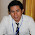I think it would be better if you provide the data in a table then put the result below the data.

1.no data, just answer the question one by one

Thank you to visit:
MATH 1280 Introduction to Statistics – Assignment Unit 4 – Part 2
-
11. Read section 4.4.1 in the book (Yakir, 2011). Do the numbers in the table above (for the random variable) represent a data sample (Yes/No)? No
It is because the Table above represents the sample space.

4.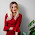1.Thank you to visit:
MATH 1280 Introduction to Statistics – Assignment Unit 4 – Part 2
-
12. In the random variable table shown above, the value in the second row represents the cumulative probability of the corresponding values in the first row (True/False) : FALSE

It is because the row represents the individual probability for each of the sample space. the probability value for the last entry is 1.

5.Interesting post :) have a lovely day :)

1.Thank you to visit:
MATH 1280 Introduction to Statistics – Assignment Unit 4 – Part 2
-
13. The probability that a randomly selected value from this random value will be less than or equal to 3 is : 0.6

Steps to find answer: P(X<=3) = 0.10+0.15+0.25+0.10 = 0.60

6.Love the paintings, Jackson Pollock is a brave man.

1.Thank you to visit:
MATH 1280 Introduction to Statistics – Assignment Unit 4 – Part 2
-
14. What is the probability that a randomly selected value from the random variable would be exactly 1.5?

7.Interesting post!

1.Thank you to visit:
MATH 1280 Introduction to Statistics – Assignment Unit 4 – Part 2
-
15. Review section 4.4 in the book (Yakir, 2011), especially pages 57—58. The expectation of the random variable is: 2.95

E(X) = 0*0.10 + 1*0.15 + 2*0.25 + 3*0.10 + 4*0.15 + 5*0.10 + 6*0.15 = 2.95

8.very clever post! Thanks for sharing!
Don't Call Me Fashion Blogger
Bloglovin'

1.Thank you to visit:
MATH 1280 Introduction to Statistics – Assignment Unit 4 – Part 2
-

9.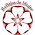Have a nice weekend

1.Thank you to visit:
MATH 1280 Introduction to Statistics – Assignment Unit 4 – Part 2
-
16. To find the expectation of a random variable by using a relative frequency table, you can add the values in the first row of the table and divide by the number of columns in the table (True/False): FALSE

Steps to find answer: We use formula to find the expectation of a random variable as of to answer question 15

10.Thanks for sharing!

Best regards

1.Thank you to visit:
MATH 1280 Introduction to Statistics – Assignment Unit 4 – Part 2
-
17. Study Yakir (2011) pp. 57-59 and solved problems 4.1.6-4.1.8. The (population) standard deviation of the random variable above is (round to 3 decimal places): 1.909

(hint, you can not put values from the table into the sd() function because the sd() function does not adjust for the probabilities).

E(X^2) = 0*0.10 + 1*0.15 + 4*0.25 + 9*0.10 + 16*0.15 + 25*0.10 + 36*0.15 = 12.35

So, Standard deviation = (E(X^2)-(E(X))^2)^0.5 = (12.35-2.952)^0.5 = 1.909

11.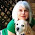Well, I am lost in math but I enjoyed so much Pollock's paintings.
They are brilliant!
Wish you a beautiful warm weekend!

1.I see...yes, Pollock is one of brilliant painters

Thank you to visit:
MATH 1280 Introduction to Statistics – Assignment Unit 4 – Part 2
-
18. If you have already calculated the standard deviation of a data sample, what is the next thing to do to find the variance:

Variance is equal to square of standard deviance

E(X^2) = 0*0.10 + 1*0.15 + 4*0.25 + 9*0.10 + 16*0.15 + 25*0.10 + 36*0.15 = 12.35

-

12.You've tangled my head ... interesting post.
Nice weekend.

1.Thank you to visit:
MATH 1280 Introduction to Statistics – Assignment Unit 4 – Part 2
-
A Random Variable:
9. The missing probability value (under the number 4) in the random variable table above is: 0.15
1 - (0.10+0.15+0.25+0.10+0.10+0.15) = 0.15

13.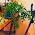nice abstracts

1.Thank you to visit:
MATH 1280 Introduction to Statistics – Assignment Unit 4 – Part 2
-
10. The sum of the probabilites in the second row of any random variable table like the one above should equal (round to 3 decimal places): 1
Based on theory that for any Random Variables, sum of probabilities should be 1 (one)

14.I know those paintings, so pretty in not obvious sense☺

1.Thank you to visit:
MATH 1280 Introduction to Statistics – Assignment Unit 4 – Part 2
-
11. Read section 4.4.1 in the book (Yakir, 2011). Do the numbers in the table above (for the random variable) represent a data sample (Yes/No)? No
It is because the Table above represents the sample space.

15.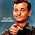Have I mentioned I suck at math?

16.Thank you to visit:
MATH 1280 Introduction to Statistics – Assignment Unit 4 – Part 2
-
12. In the random variable table shown above, the value in the second row represents the cumulative probability of the corresponding values in the first row (True/False) : FALSE